# Chi-Square Distribution

(redirected from "Chi-squared" distribution)
Also found in: Dictionary, Medical.

## chi-square distribution

[′kī ¦skwer dis·trə′byü·shən]
(statistics)
The distribution of the sum of the squares of a set of variables, each of which has a normal distribution and is expressed in standardized units.
McGraw-Hill Dictionary of Scientific & Technical Terms, 6E, Copyright © 2003 by The McGraw-Hill Companies, Inc.
The following article is from The Great Soviet Encyclopedia (1979). It might be outdated or ideologically biased.

## Chi-Square Distribution

The probability distribution of the sum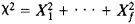of the squares of the normally distributed random variables X1, . . ., Xf, with zero mathematical expectation and unit variance is known as a chi-square distribution with f degrees of freedom. The distribution function for a chi-square random variable is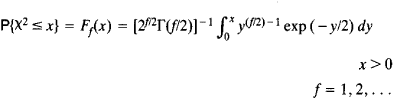The first three moments (the mathematical expectation, the variance, and the third central moment) of χ2 are f, 2f, and 8f, respectively. The sum of two independent random variables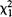and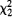with f1 and f2 degrees of freedom has a chi-square distribution with f1 + f2 degrees of freedom.

Examples of chi-square distributions are the distributions of the squares of random variables that obey the Rayleigh and Maxwellian distributions. The Poisson distribution can be expressed in terms of a chi-square distribution with an even number of degrees of freedom: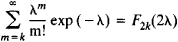If the number f of terms of the sum χ2 increases without bound, then, according to the central limit theorem, the distribution function of the standardized ratioconverges to the standard normal distribution: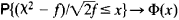where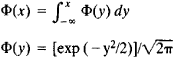A consequence of this fact is another limit relation, which is convenient for calculating Ff(x) when f has large values: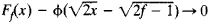In mathematical statistics, the chi-square distribution is used to construct interval estimates and statistical tests. Let Yi, . . ., Yn be random variables representing independent measurements of an unknown constant a. Suppose the measurement errors Yia are independent and are distributed identically normally. We have

E(Yia) = 0 E(Yia)2 = σ2

The statistical estimate of the unknown variance σ2 is then expressed by the equation

s2 = S2/(n – 1)

where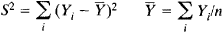The ratio S22 obeys a chi-square distribution with f = n – 1 degrees of freedom. Let x1 and x2 be positive numbers that are solutions of the equations Ff(x1) = α/2 and Ff(x2) = 1 – α/2, where α is a specified number in the interval (0,1/2). In this case

P{x1 < S22 < x2} = P{S2/x2 < σ2 < S2/x1} = 1 – α

The interval (S2/x1, S2/x2 is called the confidence interval for σ2 with confidence coefficient 1 – α.

This method of constructing an interval estimate for σ2 is often used to test the hypothesis that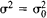, where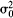is a given number. Thus, ifbelongs to the confidence interval indicated, then one concludes that the measurements do not contradict the hypothesis. If, however,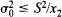or, then it must be assumed that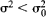or, respectively. This test corresponds to a significance level equal to α.

### REFERENCE

Cramer, H. Matematicheskie metody statistiki, 2nd ed. Moscow, 1975. (Translated from English.)

L. N. BOLSHEV